### What is called a Junction:

Materials like semiconductor, metals, insulators are joined together to form electronic devices. The interface between these materials is called the junctions. These junctions may affect the electrical properties of the materials. Ideal junctions do not affect the properties. Following are the types of junctions.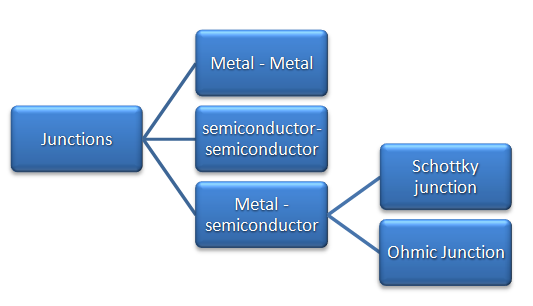Types of junctions

### Fermi level of metal and semiconductors:

Fermi level is the highest energy level occupied by the electrons in the material at absolute zero temperature. Energy level of  conduction band consists of the electrons which are responsible for conduction. These electrons jumps up from the valence shell and becomes free. The energy level of the valence band consists of the electrons in the valence shell.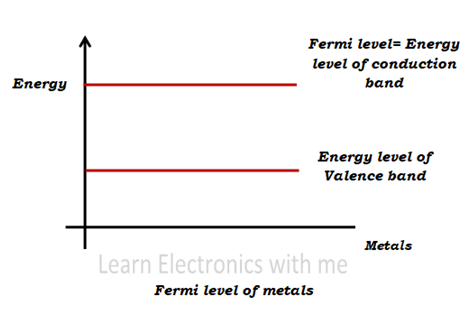Fermi level of metals

Metals are conductors and so the Fermi level and the energy level of conductors are the same.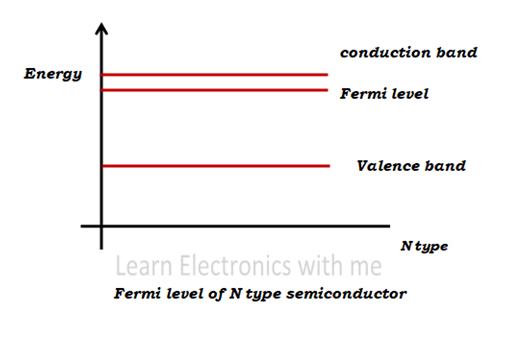Fermi level of n type semiconductor

Semiconductors do not conduct at pure form. N type semiconductors are doped with pentavalent impurity. Here the majority carriers are electrons and minority carriers are holes. Fermi level is near the conduction band.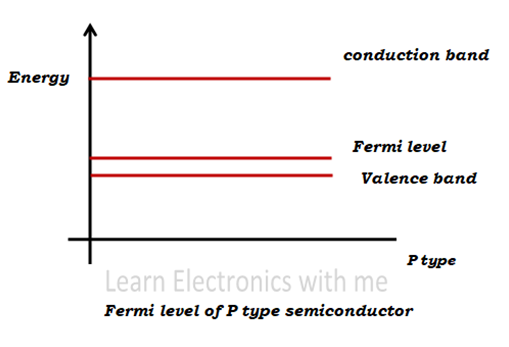Fermi level of p type semiconductor

P type semiconductors are doped with trivalent impurities. Here the majority carriers are holes and minority carriers are electrons. Fermi level is near valence band.

### What is metal semiconductor junction?

As the name says metal semiconductor junction is metals and semiconductors are joined together to form electronic devices. There are mainly two types of metal semiconductor junction

1) Schottky barrier (Φm > Φsemi)

2) Ohmic contact (Φm < Φsemi)

The distance between the Fermi level and the vacuum level is called as the work function( Φ).

First let us learn what happens when metal and semiconductor is joined and the changes in their energy levels.

### Energy band diagram before metal- semiconductor contact: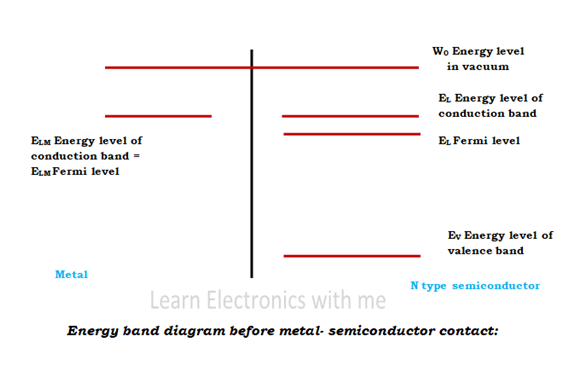Energy band diagram before metal semiconductor contact

The above figure shows the Fermi level, energy level of conduction and valence band of metals and semiconductor before they are joined.

### Energy band diagram after metal- semiconductor contact: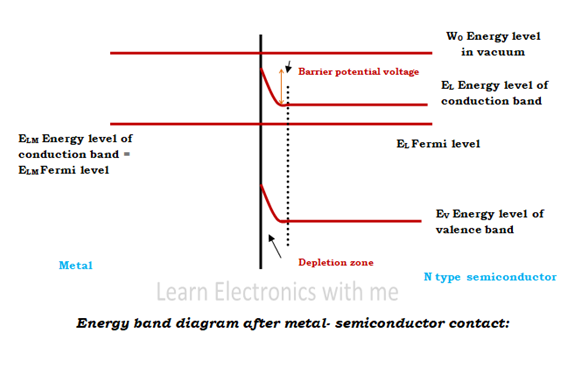Energy band diagram after metal semiconductor contact

When the metal and the semiconductor come in contact, the Fermi level of both metal and semiconductor tend to become equal. The energy level of conduction band of the semiconductor is higher than the Fermi level. So the electrons from higher energy level tend to flow to lower energy level. So the electrons in the conduction band decreases and the distance between the Fermi level and the energy level of conduction band decreases.

The electrons which had moved from higher energy level to lower energy level leaves holes behind which are positive ions. And thus the depletion zone is formed and the width of the depletion zone depends on the amount of impurity added.

### 1) Schottky barrier: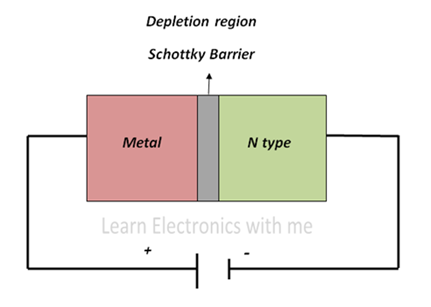Schottky barrier

Depletion layer is formed in the semiconductor due to the transfer of electrons from semiconductor to metal. Thus the Schottky barrier is formed at the junction of N type semiconductor and the metal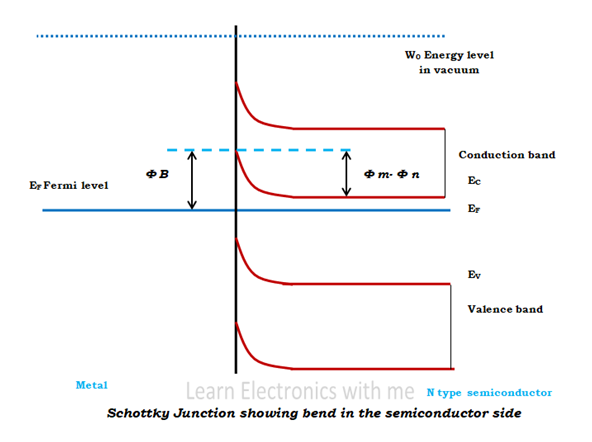Energy band diagram of schottky barrier

The depletion layer formed in the semiconductor extends and thus the bend is formed in the energy band diagram of semiconductor. From the above figure we observe that work function ΦM of metal is larger than than the work function of the semiconductor ΦN. There is a built in potential formed in the schottky barrier. It is given by

eV0 = Φm – Φsemi

The work function of the metal remains constant but the work function of the semiconductor depends on the doping concentration. The barrier which is formed prevents the electrons to move from metal to semiconductor and from semiconductor to metal. The schottky barrier is denoted by ΦB and is given by

ΦB= Φm-Xn, where Xn is the electron affinity of n type semiconductor. There are two types of bias applied to the schottky barrier, they are

a) Forward bias

b) Reverse bias

#### a) Forward bias

When external voltage is applied, when forward biased the positive terminal is connected to the metal and the negative terminal is connected to the N type semiconductor. The electrons receives more energy to cross the junction barrier and move from N type semiconductor to the metal and thus the current starts to flow. The current is due to the drift of majority charge carriers. Since there is no P type semiconductor there is no holes and thus no minority carriers.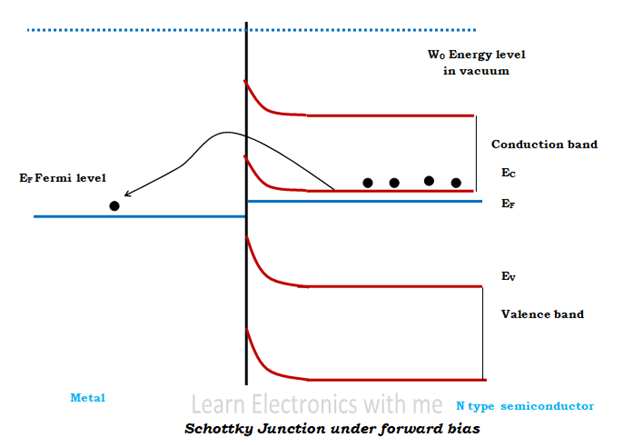Schottky junction at forward bias

From the above figure we observe that when forward biased the Fermi level of the metal is lower than the Fermi level of semiconductor. So the schottky barrier ΦB decreases across the semiconductor which makes electrons to diffuse easily from semiconductor to metal. Because of this movement of electrons, a positive current is formed across the junction.

#### b) Reverse bias

When reverse biased the positive terminal is connected to the N type semiconductor and the negative terminal is connected to the metal. The size of the depletion region increases and the current stops to flow. There is small amount of leakage current. When the applied voltage is increased further the current increases and when increased further the depletion region breaks down which damages the device permanently.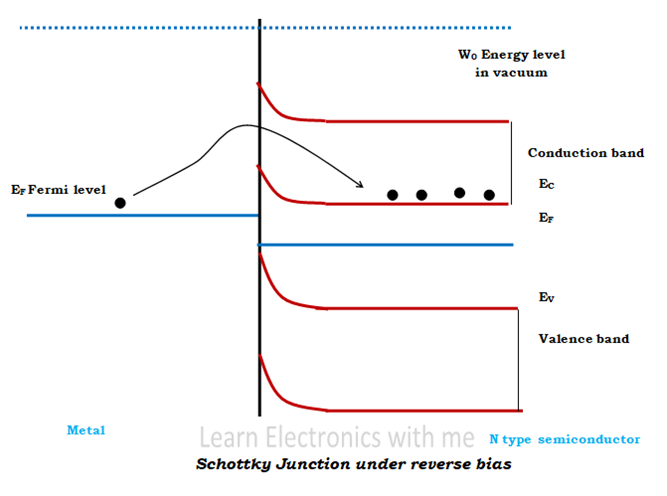Schottky junction at reverse bias

When we observe from the above figure the Fermi level of the metal is higher than the Fermi level of the semiconductor. So the potential across the barrier increases and blocks the electrons from diffusing from semiconductor to metal.

### 2) Ohmic contact:

Potential barrier is not formed at all cases when metal and semiconductor is joined. So in that case when no potential barrier is formed, it is called as Ohmic contact or Ohmic junction.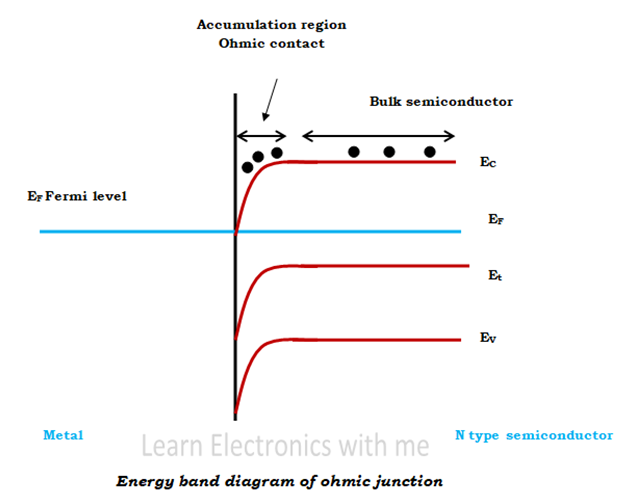Energy band diagram of ohmic junction

Here the work function of metal Φm is lesser than the work function of semiconductor Φsemi. The electrons move from metal to semiconductor and thus the Fermi level of the semiconductor moves up till equilibrium state is established. Since there is no barrier even a small forward bias will produce large forward bias current and when reverse bias is applied small barrier is formed but it is removed when the reverse bias is increased further.

### V-I characteristics of Schottky barrier and Ohmic contact: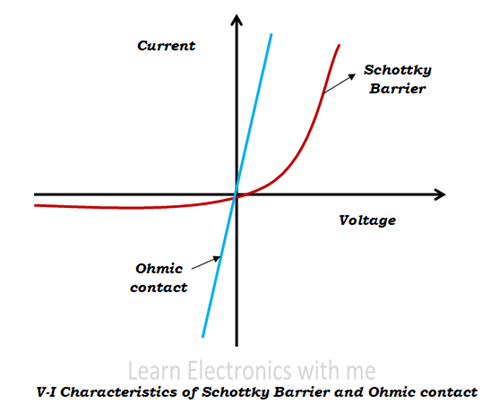V-I characteristics of Schottky barrier and ohmic contact

From the above figure we observe that in the schottky barrier during forward bias the current increases after the electrons overcomes the potential barrier at the junction and during reverse bias the depletion layer increases and thus only very small amount of current flows.

In the Ohmic contact since there is no potential barrier, small amount of bias produces large current in both forward and reverse bias.